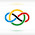### 2020 IMO #3

There are $4n$ pebbles of weights $1, 2, 3, \dots, 4n.$ Each pebble is coloured in one of $n$ colours and there are four pebbles of each colour. Show that we can arrange the pebbles into two piles so that the following two conditions are both satisfied:

1) The total weights of both piles are the same.

2) Each pile contains two pebbles of each colour.

Note that the sum of numbers of a pile is $n(4n+1)$.

We will form one such pile by by adding up pairs of numbers which sum to $4n+1$ , keeping two number of the same color. The other pile follows immediately.

Consider the (possibly not simple) graph $\mathcal G$ on $n$ vertices with each vertex representing a color. For every $k \in \{1,2\dots, 4n+1\}$, we will draw an edge between the color of $k$ and the color of $4n+1-k$. Some observations are :

Every vertex has degree $4$.

The total number of edges in every connected component are hence even

Note that due to the second observation, a Eulerian circuit exists. Pick every alternate edge in the Eulerian circuit and put them in the pile. After this process, we would have a pile with exactly $2n$ stones since half the edges are chosen. Furthermore, every color is picked twice, since of the four edges emanating from each vertex, exactly two are chosen since we alternate which one we add.

We are done. $\square$

1.Wow!

I loved your solution, and I especially admire the insight of drawing an edge between k and 4n+1-k.

Good job.

2.Thanks!